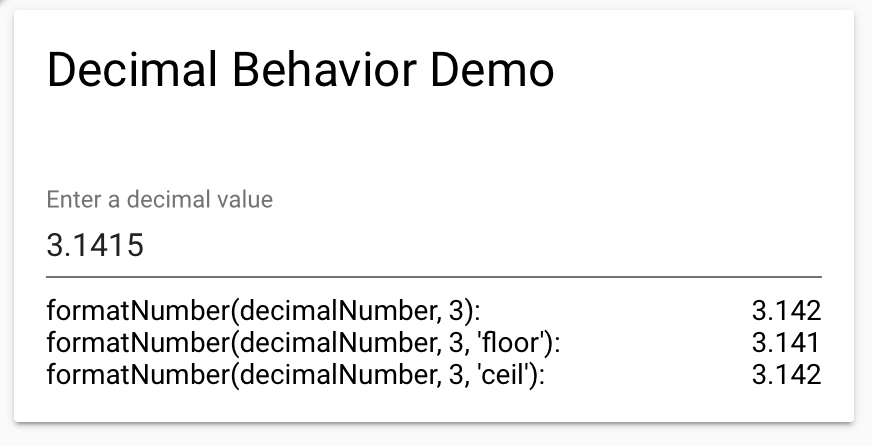Subscription# Directory

← Back

## decimal-behavior

A decimal-behavior element that provides ceil, round, floor, format and decimalAdjustemnt methods. Decimal adjustment of a number implementation is taken from Mozilla Developer Network: https://developer.mozilla.org/en-US/docs/Web/JavaScript/Reference/Global_Objects/Math/round.

### Rating[ This description is mirrored from README.md at github.com/Protoss78/decimal-behavior on 2019-05-10 ]# decimal-behavior

A decimal-behavior element that provides ceil, round, floor, format and decimalAdjustemnt methods. Decimal adjustment of a number implementation is taken from Mozilla Developer Network: https://developer.mozilla.org/en-US/docs/Web/JavaScript/Reference/Global_Objects/Math/round.

API documentation and live demo can be found on the component page

## Installation

Install using bower

``````bower i decimal-behavior -S
``````

Import the behavior

``````<link rel="import" href="../bower_components/decimal-behavior/decimal-behavior.html">
``````

Inlude the behavior in your web component

``````behaviors: [DecimalBehavior]
``````

## Example

``````<dom-module id="x-decimal-behavior">
<template>
<style>
:host {
display: inline-block;
}
</style>
<h1>decimal-behavior</h1>
<div>round: [[formatNumber(3.125, 2)]]</div>
<div>floor: [[formatNumber(3.1415, 3, 'floor')]]</div>
<div>ceil : [[formatNumber(3.14151, 4, 'ceil')]]</div>
</template>
<script>
Polymer({
is: 'x-decimal-behavior',
behaviors: [DecimalBehavior]
});
</script>
</dom-module>
<x-decimal-behavior></x-decimal-behavior>
``````

## Methods

### round(value, exp)

Decimal adjustment of a number using the round method.

• value: The number to be adjusted
• exp: The exponent (the 10 logarithm of the adjustment base). -1 = round to 1 decimal

### floor(value, exp)

Decimal adjustment of a number using the floor method.

• value: The number to be adjusted
• exp: The exponent (the 10 logarithm of the adjustment base). -1 = round to 1 decimal

### ceil(value, exp)

Decimal adjustment of a number using the ceil method.

• value: The number to be adjusted
• exp: The exponent (the 10 logarithm of the adjustment base). -1 = round to 1 decimal

### formatNumber(value, decimals, type)

Adjusts and formats the passed value to the specified number of decimals using the passed adjustment type. The toFixed function is used to cut off the String representation.

• value: The number to be adjusted
• decimals: The number of decimals (is converted into an exponent). Example 1 = 1 decimal position
• type: The adjustment type: round, floor or ceil. round is set as default.

Decimal adjustment of a number. Original implementation from Mozilla Developer Network: https://developer.mozilla.org/en-US/docs/Web/JavaScript/Reference/Global_Objects/Math/round

• type: The adjustment type: round, floor or ceil
• value: The number to be adjusted
• exp: The exponent (the 10 logarithm of the adjustment base). -1 = round to 1 decimal

### Dependencies

• polymer#Polymer/polymer#1.9 - 2
Released
2017-05-30
Maturity
IMPORTED